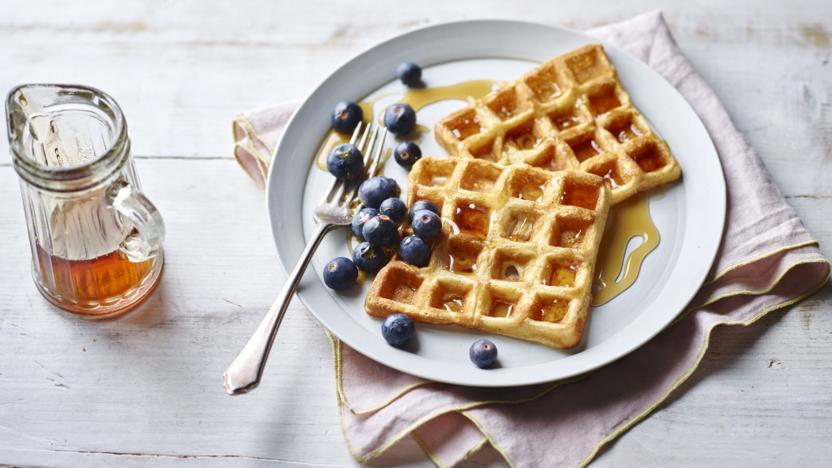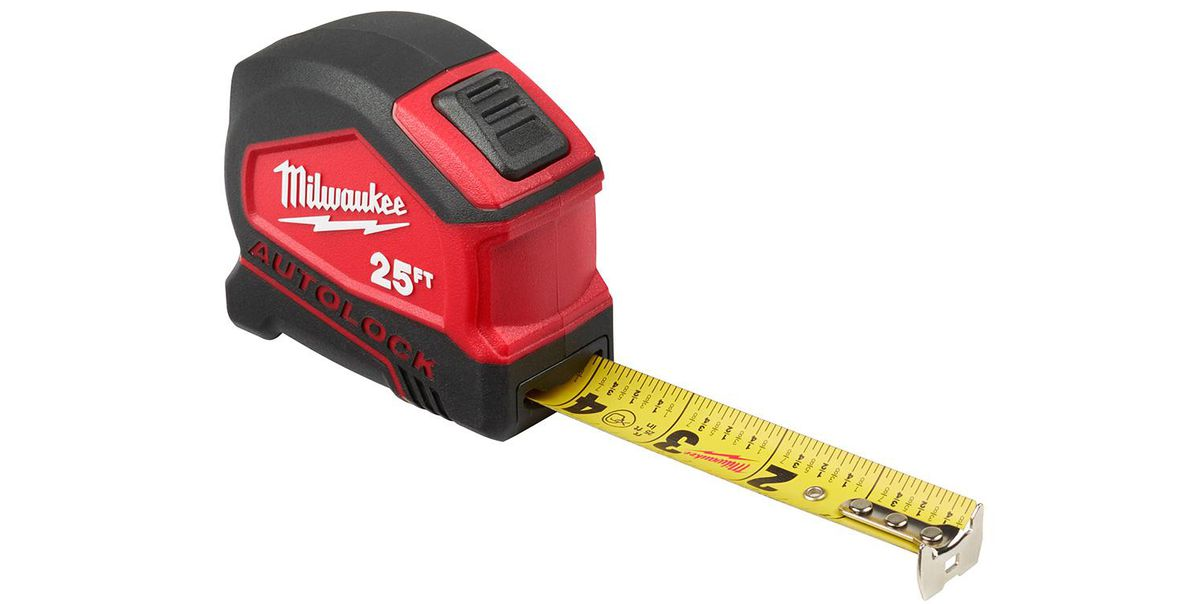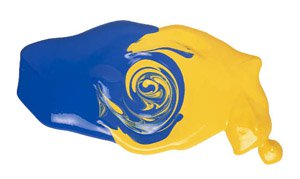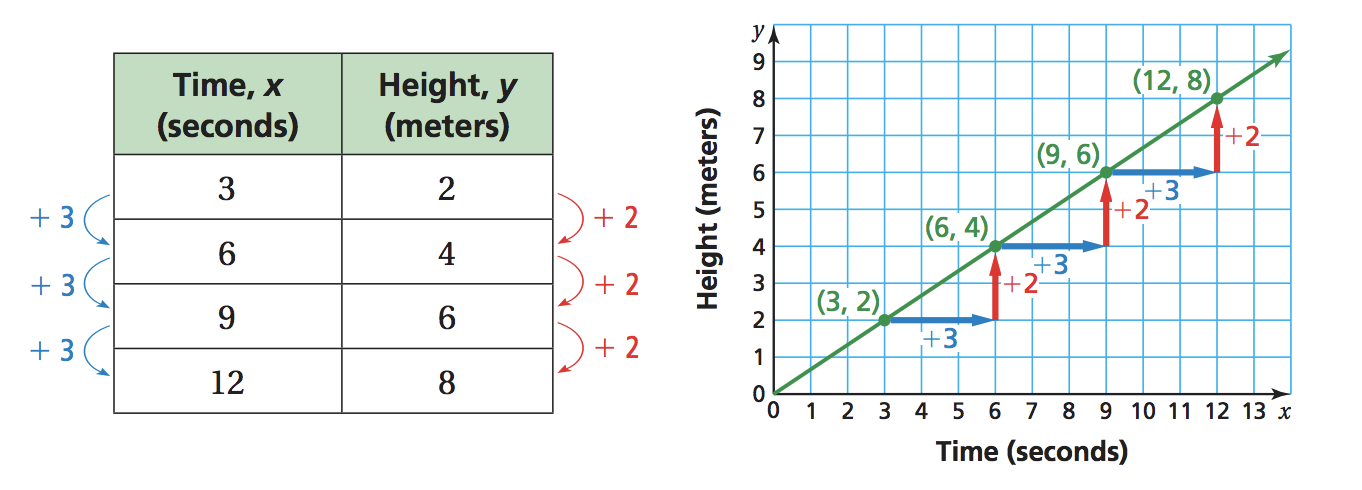Ratios, Rates, and Proportions
starstarstarstarstarstarstarstarstarstar
by Felix Gabathuler
| 27 Questions1
1
In your own words, what is a ratio? How would you explain that concept to someone? Can you use an example to make your point?2
1
Waffles are made with 2½ cups water and 1½ cups milk. What is the ratio of water to milk? Write your answer using a colon.
3
1
Directions: Use the whole numbers 0 through 9, at most one time fill in the boxes below to create an accurate number line. Imagine the line is to scale, so pick your numbers based on where you think they fall on the number line.4
1
Why do you think rates are usually written as unit rates? Use an example to support your opinion.
5
1
A school bus drove 2½ hours to a football game. If the bus averaged 35 mph, how far did it travel? (Psst... distance = (speed)(time). ((Double Pssst: Don't forget units...))
6
1
Mr. Kevin pays \$37.95 per month for his cell phone, with unlimited minutes. At this rate, what will his cell phone cost for the year?7
1
If 1 in = 2.54 cm and 1ft = 12 in, then 1 ft = _____  cm?
8
1
Franky orders 8 reams of custom stationary, where a ream is 500 sheets of paper. If the order costs \$11.05 per ream, and each sheet is 8½ inches by 11 inches long, what is the total cost of the order?
9
1
A person reads a books at a rate of 1 word every 0.0145 seconds. How long will it take to read 3,500  words? (psst... don't forget units...)
10
1
A leak in a storage tank caused 600  gallons of water to drain out in 70  minutes. What is the average rate of change in the tank water level?
11
1
Fuffy spent \$7.20 on 4 dozen pencils. How much did each pencil cost?12
1
For our math mural, Mr. Robert has to mix 2/5 cup of red paint for every 1/4 cup of blue paint to make 1 and 5/8 gallons of purple paint. (Hint - there are 16 cups in 1 gallon)

How much red paint did he use?
13
1
For our math mural, Mr. Robert has to mix 2/5 cup of red paint for every 1/4 cup of blue paint to make 1 and 5/8 gallons of purple paint. (Hint - there are 16 cups in 1 gallon)

How much blue paint did he use?14
1
Now let's discuss and practice the concept of a proportion. Can you explain what a proportion is? Try to use relevant math vocab to strengthen your definition.
15
1
Select the pairs that are proportional:

16
1
Does the following scenario represent a proportion: 120 units made in 5 days; 88 units made in 4 days. Answer yes or no, then explain how you know.
17
1
Which proportion is equivalent to wx = yz ?

Watch the video below to get a better feel of what a proportional relationship is and how it can look. Rewrite your definition above if needed, especially when using tables.
Here's another great video that demonstrates how to tell if something is a proportion. Give it a watch so you can answer the questions that follow!
18
1
Now use your math skillz, and maybe your notes too, to determine mathematically if things are proportional.

Determining Proportionality Mathematically
Yup
Nope
19
1
Alright, my brain hurts... can we do something simpler? Like maybe solving proportions..? YES! Here's a present for your brain! Enjoy!

Solving Proportions

Watch the video below if you have some problems with the Solving Proportions. If not, carry on!
Solved
Not solvedGiven a table of ratios, you can transfer the data to a graph. Look at the table below and how it translates into a straight line on the graph.The structure in the ratio table shows why the graph has a constant rate of change (write that in your notes... right now. Mr. Felix is secretly watching you from the canteen). The structure in the ratio table shows why the graph has a constant rate of change.
20
1
Use a graph to tell whether x and y are in a proportional relationship. Plot the given table on the graph provided. Is it a straight line? Is it proportional?21
1
Interpret each plotted point in the graph of the proportional relationship.

22
1
The graph of a proportional relationship passes through (12, 16) and (1, y). Find y.23
1
A rough rule for finding the correct bat length is “the bat length should be half of the batter’s height.” So, a 62-inch-tall batter uses a bat that is 31 inches long. Write a proportion to find the bat length for a batter that is 58 inches tall.

24
1
The batting average of a baseball player is the number of “hits” divided by the number of “at bats.”

Write a proportion to find how many hits H a player needs to achieve if he is 50 times at bat with a batting average of 0.200.
25
1
Solve the proportion:

26
1
How did you solve the problem above?27
1
What is the fewest number of people surveyed if exactly 93.6% of people completed a survey?